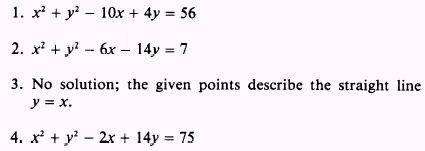Custom SearchPRACTICE PROBLEMS: Find the coordinates of the center and the radius for the circles described by the following equations: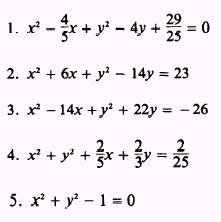ANSWERS: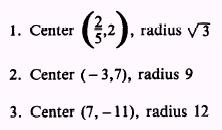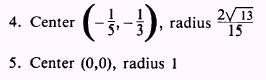In certain situations you will want to consider the following general form of a circle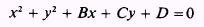as the equation of a circle in which the specific values of the constants B, C, and D are to be determined. In this problem the unknowns to be found are not x and y, but the values of the constants B, C, and D. The conditions that define the circle are used to form algebraic relationships between these constants. For example, if one of the conditions imposed on the circle is that it pass through the point (3,4), then the general form is written with x and y replaced by 3 and 4, respectively; thus,is rewritten as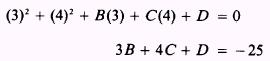Three independent constants (B, C, and D) are in the equation of a circle; therefore, three conditions must be given to define a circle. Each of these conditions will yield an equation with B, C, and D as the unknowns. These three equations are then solved simultaneously to determine the values of the constants, which satisfy all of the equations. In an analysis, the number of independent constants in the general equation of a curve indicate how many conditions must be set before a curve can be completely defined. Also, the number of unknowns in an equation indicates the number of equations that must be solved simultaneously to find the values of the unknowns. For example, if B, C, and D are unknowns in an equation, three separate equations involving these variables are required for a solution. A circle may be defined by three noncollinear points; that is, by three points not lying on a straight line. Only one circle is possible through any three noncollinear points. To find the equation of the circle determined by three points, substitute the x and y values of each of the given points into the general equation to form three equations with B, C, and D as the unknowns. These equations are then solved simultaneously to find the values of B, C and D in the equation which satisfies the three given conditions. The solution of simultaneous equations involving two variables is discussed in Mathematics, Volume 1. Systems involving three variables use an extension of the same principles, but with three equations instead of two. Step-by-step explanations of the solution are given in the example problems. EXAMPLE: Write the equation of the circle that passes through the points (2,8), (5,7), and (6,6). SOLUTION.- The method used in this solution corresponds to the addition-subtraction method used for solution of equations involving two variables. However, the method or combination of methods used depends on the particular problem. No single method is best suited to all problems. First, write the general form of a circle:For each of the given points, substitute the given values for x and y and rearrange the terms: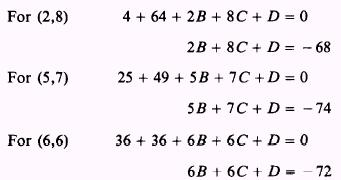To aid in the explanation, we number the three resulting equations: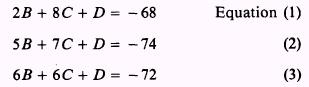The first step is to eliminate one of the unknowns and have two equations and two unknowns remaining. The coefficient of D is the same in all three equations and is, therefore, the one most easily eliminated by addition and subtraction. To eliminate D, subtract equation (2) from equation (1):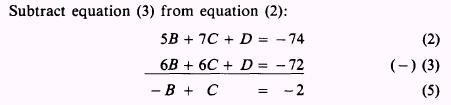We now have two equations, (4) and (5), in two unknowns that can be solved simultaneously. Since the coefficient of C is the same in both equations, it is the most easily eliminated variable. To eliminate C, subtract equation (4) from equation (5):To find the value of C, substitute the value found for B in equation (6) in equation (4) or (5)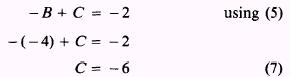Now the values of B and C can be substituted in any one of the original equations to determine the value of D. If the values are substituted in equation (1),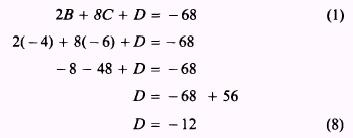The solution of the system of equations gave values for three independent constants in the general equationWhen the constant values are substituted, the equation takes the form of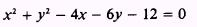Now rearrange and complete the square in both x and y: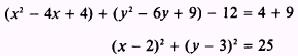The equation now corresponds to a circle with its center at (2,3) and a radius of 5. This is the circle passing through three given points, as shown in figure 2-7, view A. The previous example problem showed one method we can use to determine the equation of a circle when three points are given. The next example shows another method we can use to solve the same problem. One of the most important things to keep in mind when you study analytic geometry is that many problems may be solved by more than one method. Each problem should be analyzed carefully to determine what relationships exist between the given data and the desired results of the problem. Relationships such as distance from one point to another, distance from a point to a line, slope of a line, and the Pythagorean theorem will be used to solve various problems.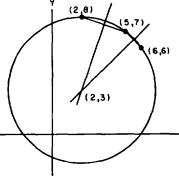Figure 2-7.-Circle described by three points. EXAMPLE: Find the equation of the circle that passes through the points (2,8), (5,7), and (6,6). Use a method other than that used in the previous example problem. SOLUTION: A different method of solving this problem results from the reasoning in the following paragraphs: The center of the desired circle will be the intersection of the perpendicular bisectors of the chords connecting points (2,8) with (5,7) and (5,7) with (6,6), as shown in figure 2-7, view B. The perpendicular bisector of the line connecting two points is the locus of all points equidistant from the two points. Using this analysis, we can get the equations of the perpendicular bisectors of the two lines. Equating the distance formulas that describe the distances from the center, point (x,y), which is equidistant from the points (2,8) and (5,7), gives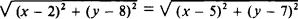Squaring both sides givesor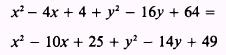Canceling and combining terms results in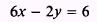or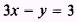Follow the same procedure for the points (5,7) and (6,6):Squaring each side gives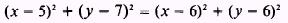or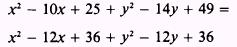Canceling and combining terms gives a second equation in x and y: 2x-2y= - 2 orSolving the equations simultaneously gives the coordinates of the intersection of the two perpendicular bisectors; this intersection is the center of the circle.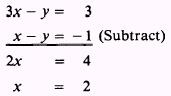Substitute the value x = 2 in one of the equations to find the value of y: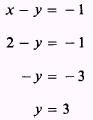Thus, the center of the circle is the point (2,3). The radius is the distance between the center (2,3) and one of the three given points. Using point (2,8), we obtain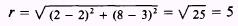The equation of this circle isas was found in the previous example. If a circle is to be defined by three points, the points must be noncollinear. In some cases the three points are obviously noncollinear. Such is the case with the points (1, 1), ( - 2,2), and (- 1, - 1), since these points cannot be connected by a straight line. However, in many cases you may find difficulty determining by inspection whether or not the points are collinear; therefore, you need a method for determining this analytically. In the following example an attempt is made to find the circle determined by three points that are collinear. EXAMPLE: Find the equation of the circle that passes through the points (1, 1), (2,2), and (3,3). SOLUTION: Substitute the given values of x and y in the general form of the equation of a circle to get three equations in three unknowns:To eliminate D, first subtract equation (1) from equation (2):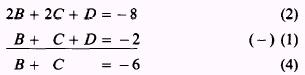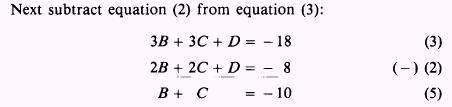Then subtract equation (5) from equation (4) to eliminate one of the unknowns: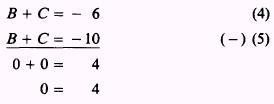This solution is not valid, so no circle passes through the three given points. You should attempt to solve equations (4) and (5) by the substitution method. When the three given points are collinear, an inconsistent solution of some type will result. If you try to solve the problem by eliminating both B and C at the same time (to find D), another type of inconsistent solution results. With the given coefficients you can easily eliminate both A and B at the same time. First, multiply equation (2) by 3 and equation (3) by - 2 and add the resultant equations: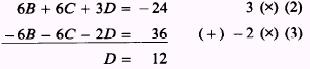Then multiply equation (1) by - 2 and add the resultant to equation (2):This gives two values for D, which is inconsistent since each of the constants must have a unique value consistent with the given conditions. The three points are on the straight line y = x. PRACTICE PROBLEMS: In each of the following problems, find the equation of the circle that passes through the three given points: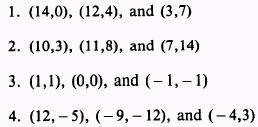ANSWERS: